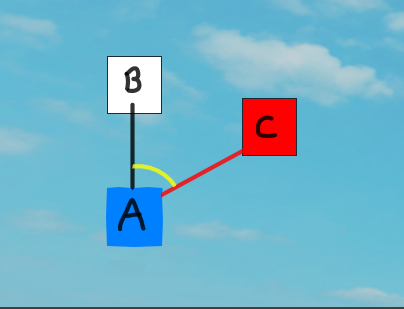# How do I find the angle between 3 GUI elements

greetings. i have tried so much math and research and i am certified going bananzasI have 3 gui elements (or 3 GUI positions). The position of the red gui object C may be changed to any position. I am trying to find a formula to find the measure of the yellow angle in degrees (the angle between AB and AC) when C may be at any position in a 360* range around AB.

Ha ha! Something I can answer finally.

So it seems you want an angle, but I’m not sure if you want it signed, so I’ll give you both solutions.

Here is some magic code:

``````local aToB = (b.AbsolutePosition-a.AbsolutePosition).Unit
local aToC = (c.AbsolutePosition-a.AbsolutePosition).Unit
local closestAngle = math.acos(math.clamp(aToB:Dot(aToC), -1, 1))
local signedAngle = closestAngle * math.sign(Vector3.new(0,0,1):Dot(aToB:Cross(aToC)))
``````

aToB is the unit vector going from a to b
aToC is the unit vector going from a to c
closestAngle is basically the angular distance between the two vectors (So the positive vector between them)
signedAngle is also the closest vector, but signed, meaning that it retains the information of the direction in which it’s spinning (clockwise or counterclockwise)

Let me know if you have any questions c:<

EDIT: I’m also gonna plug here my VectorUtils, which is a library of math stuff I’ve build in the past 4 years. It contains the functions VectorUtils.AngleBetween(vector1, vector2) and VectorUtils.AngleBetweenSigned(vector1, vector2, axisOfRotation), which juuust so happen to be what you asked for.

Thank you! That sure gives me a number!

The signedAngle line gives me the error “Vector3 expected, got number”, but I don’t know if I need the signed angle

Do you know how I can get the angle in degrees or radians? I forgot to put that in the original post

Thank you so much for the help!

I’ve figured something out! A major problem was that I was putting the equation in the Wrong Part Of The Code so it kept giving me errors or weird answers that didn’t make sense and I assumed I was doing the math wrong

``````		local AB = (script.Parent.Parent.B.AbsolutePosition - script.Parent.Parent.A.AbsolutePosition)
local AC = (script.Parent.Parent.C.AbsolutePosition - script.Parent.Parent.A.AbsolutePosition)
local angle = math.acos( (AB:Dot(AC)) / (AB.magnitude * AC.magnitude))
``````

In the scenario described in the main post, this will get the angle between the two vectors in radians (unless I’m mistaken but it seems to be working from testing). The value will always be positive and below 180 degrees, so something else will have to be tacked on to find if C is positioned to the left or right of AB

Thank you so much for the help, I’ll definitely look at your library!

To find if it’s left or right, use the signedAngle instead!

1 Like